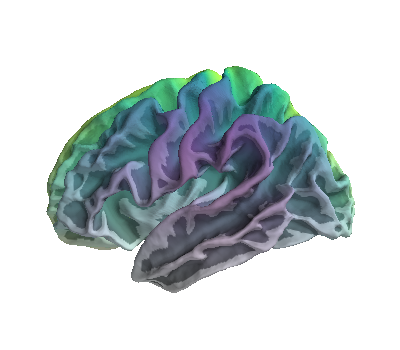# Plot RGBA values on brain surface¶

In this example, each vertex on a 3D brain is plotted with a different RGBA value. Hue varies along the x-axis (right/left direction) and alpha varies along the z-axis (up/down direction). However, this can be easily generalised to other use cases.```import os
import numpy as np
import matplotlib.pyplot as plt
from mayavi import mlab
from tvtk.api import tvtk
from tvtk.common import configure_input_data
from surfer import Brain

print(__doc__)

# 1) define helper functions

def norm(x):
'''Normalise array betweeen 0-1'''
return (x - np.min(x)) / (np.max(x) - np.min(x))

# 2) init brain and get spatial co-ordinates

# params
subjects_dir = os.environ['SUBJECTS_DIR']
hemi = 'lh'
surf = 'white'

# init figure
fig = mlab.figure()
b = Brain('fsaverage', hemi, surf, subjects_dir=subjects_dir,
background='white', figure=fig)

# co-ordinates
x, y, z = b.geo[hemi].coords.T
tris = b.geo[hemi].faces

# 3) generate an rgba matrix, of shape n_vertices x 4

# define color map
cmap = plt.cm.viridis

# change colour based on position on the x axis
hue = norm(x)
colors = cmap(hue)[:, :3]

# change alpha based on position on the z axis
alpha = norm(z)

# combine hue and alpha into a Nx4 matrix
rgba_vals = np.concatenate((colors, alpha[:, None]), axis=1)

# 4) add data to plot

# plot points in x,y,z
mesh = mlab.pipeline.triangular_mesh_source(
x, y, z, tris, figure=fig)
mesh.data.point_data.scalars.number_of_components = 4  # r, g, b, a
mesh.data.point_data.scalars = (rgba_vals * 255).astype('ubyte')

# tvtk for vis
mapper = tvtk.PolyDataMapper()
configure_input_data(mapper, mesh.data)
actor = tvtk.Actor()
actor.mapper = mapper